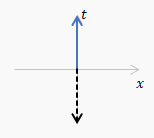## Friday, 2 July 2021

### 2.5 Differential formsVector $U$ (solid) and1-form $\widetilde{U}$ (dashed)
In section 2.5 of Misner, Thorne, Wheeler 'differential forms' or '1-forms' are introduced and there is soon a fairly elaborate recipe for constructing 1-forms $\widetilde{U}$ from a vector $U$. I reproduce it below. I tried out the recipe on some of the sample vectors shown and it seemed to work. A one form $\widetilde{U}$ is shown as a set of planes, whose spacing is defined, and which have a positive sense, or direction, as shown by a dashed arrow (which is not a vector). It would seem that you could equally well show the 1-form by a dashed arrow perpendicular (in the Euclidean sense) to the planes whose direction and length would show the positive sense and the spacing of the planes of $\widetilde{U}$. You can. And I implemented it in a spreadsheet and made the amusing gif on the right. Then I saw the joke.

### The recipe

Figure 2.7. Several vectors, $A,B,C,D,E$, and corresponding 1-forms $\widetilde{A},\widetilde{B},\widetilde{C},\widetilde{D},\widetilde{E}$. The process of drawing $\widetilde{U}$ corresponding to a given vector $U$ is quite simple. 1) Orient the surfaces of $\widetilde{U}$ orthogonal to the vector $U$ . (Why? Because any vector $V$ that is perpendicular to $U$ must pierce no surfaces of $\widetilde{U}$ ($0=U\bullet V=U,V$) and must therefore lie in a surface of $\widetilde{U}$.) 2) Space the surfaces of  $\widetilde{U}$ so the number of surfaces pierced by some arbitrary vector $Y$ (e.g., $Y=U$) is equal to $Y\bullet U$.

Note that in the figure the surfaces of $\widetilde{B}$ are, indeed, orthogonal to $B$; those of $\widetilde{C}$ are, indeed, orthogonal to $C$, etc. If they do not look so, that is because the reader is attributing Euclidean geometry, not Lorentz geometry, to the spacetime diagram. He should recall, for example, that because $C$ is a null vector, it is orthogonal to itself ($C\bullet C=0$), so it must itself lie in a surface of the 1-form  $\widetilde{C}$. Confused readers may review spacetime diagrams in a more elementary text, e.g., Taylor and Wheeler (1966)."

Read it here, including punchline: 2.5 Differential forms.pdf. (6 pages)

#### 1 comment:

1.I discovered a new simple time formula. As it is known, every simple
physics formula explains a law of nature. My formula says, ‘In a
physical event there is always as much time as the energy amount. That
is, “The more energy there is, the more time there is.'’ And vice
versa. Please can you evaluate my website and
articles? my website timeflow.org and three articles,
https://magneticuniverse.com/discussion/307/timeflow-theory-by-salih-kircalar

Special Relativity Theory (SRT) equations revealed that mass and time are
proportional.
t / t' = m / m'

My 'Timeflow'='Time'/'Energy' Formula makes a small but important
contribution to this. It expresses that the relation of mass to time is equal
to the amount of mc2 energy of the mass, which is 9x10'16 s or
2,851,927,903.26... years for 1 kg of mass outside the gravitational fields.
Very small free particles in space must be observed by organizations such as
NASA or ESA, only then my proposal will be verified.

In addition,The flow of the thought energy intensity in our brain is
body pain, unhappiness and boredom, joy, happiness and love,
sleep, and finally death, respectively, from high energy to low
energy. At the moment
to sleep, if we had a good sleep, our thought energy is very close to
zero or zero. When the energy flow intensity increases in our brain,
according to the 'Timeflow Formula (Timeflow=Time/Energy). The
timeflow will slow down. As the energy density (power) decreases, the
timeflow will accelerate. In the case of sleep and death, the timeflow
will be infinite. The timeflow formula explains very clearly and
simply that this situation, which is perceived as psychological time
is actually a purely physical event. I think it would be very useful
for psychology experts to evaluate the 'Timeflow' Formula and the
philosophical interpretation of the formula.